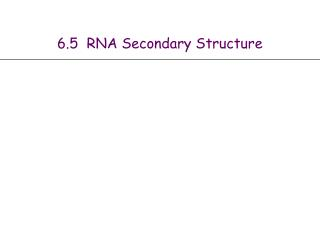DownloadDownload Presentation6.5 RNA Secondary Structure

# 6.5 RNA Secondary Structure

Download Presentation## 6.5 RNA Secondary Structure

- - - - - - - - - - - - - - - - - - - - - - - - - - - E N D - - - - - - - - - - - - - - - - - - - - - - - - - - -
##### Presentation Transcript

1. 6.5 RNA Secondary Structure

2. RNA Secondary Structure • RNA. String B = b1b2bn over alphabet { A, C, G, U }. • Secondary structure. RNA is single-stranded so it tends to loop back and form base pairs with itself. This structure is essential for understanding behavior of molecule. C A Ex: GUCGAUUGAGCGAAUGUAACAACGUGGCUACGGCGAGA A A A U G C C G U A A G G U A U U A G A C G C U G C G C G A G C G A U G complementary base pairs: A-U, C-G

3. RNA Secondary Structure • Secondary structure. A set of pairs S = { (bi, bj) } that satisfy: • [Watson-Crick.] S is a matching and each pair in S is a Watson-Crick complement: A-U, U-A, C-G, or G-C. • [No sharp turns.] The ends of each pair are separated by at least 4 intervening bases. If (bi, bj)  S, then i < j - 4. • [Non-crossing.] If (bi, bj) and (bk, bl) are two pairs in S, then we cannot have i < k < j < l. • Free energy. Usual hypothesis is that an RNA molecule will form the secondary structure with the optimum total free energy. • Goal. Given an RNA molecule B = b1b2bn, find a secondary structure S that maximizes the number of base pairs. approximate by number of base pairs

4. RNA Secondary Structure: Examples • Examples. G G G G G G G C U C U G C G C U C A U A U A G U A U A U A base pair U G U G G C C A U U G G G C A U G U U G G C C A U G A A A 4 ok sharp turn crossing

5. RNA Secondary Structure: Subproblems • First attempt. OPT(j) = maximum number of base pairs in a secondary structure of the substring b1b2bj. • Difficulty. Results in two sub-problems. • Finding secondary structure in: b1b2bt-1. • Finding secondary structure in: bt+1bt+2bn-1. match bt and bn t n 1 OPT(t-1) need more sub-problems

6. Dynamic Programming Over Intervals • Notation. OPT(i, j) = maximum number of base pairs in a secondary structure of the substring bibi+1bj. • Case 1. If i  j - 4. • OPT(i, j) = 0 by no-sharp turns condition. • Case 2. Base bj is not involved in a pair. • OPT(i, j) = OPT(i, j-1) • Case 3. Base bj pairs with bt for some i  t < j - 4. • non-crossing constraint decouples resulting sub-problems • OPT(i, j) = 1 + maxt { OPT(i, t-1) + OPT(t+1, j-1) } • Remark. Same core idea in CKY algorithm to parse context-free grammars. take max over t such that i  t < j-4 andbt and bj are Watson-Crick complements

7. Bottom Up Dynamic Programming Over Intervals • Q. What order to solve the sub-problems? • A. Do shortest intervals first. • Running time. O(n3). RNA(b1,…,bn) { for k = 5, 6, …, n-1 for i = 1, 2, …, n-k j = i + k Compute M[i, j] return M[1, n] } 4 0 0 0 3 0 0 i 0 2 1 6 7 8 9 using recurrence j

8. Dynamic Programming Summary • Recipe. • Characterize structure of problem. • Recursively define value of optimal solution. • Compute value of optimal solution. • Construct optimal solution from computed information. • Dynamic programming techniques. • Binary choice: weighted interval scheduling. • Multi-way choice: segmented least squares. • Adding a new variable: knapsack. • Dynamic programming over intervals: RNA secondary structure. • Top-down vs. bottom-up: different people have different intuitions. Viterbi algorithm for HMM also usesDP to optimize a maximum likelihoodtradeoff between parsimony and accuracy CKY parsing algorithm for context-freegrammar has similar structure